NEET  >  Previous Year Questions (2014-22): Motion in a Straight Line

# Previous Year Questions (2014-22): Motion in a Straight Line - Notes | Study Physics Class 11 - NEET

 1 Crore+ students have signed up on EduRev. Have you?

Q.1. The displacement-time graphs of two moving particles make angles of 30° and 45° with the x-axis as shown in the figure. The ratio of their respective velocity is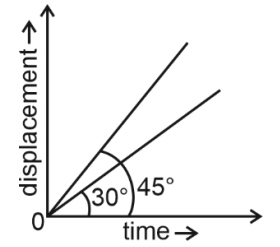(1) 1 : 1
(2) 1 : 2
(3) 1: √3
(4) √3 : 1

Ans: (3)
Solution:

Slope of x-t curves gives the velocity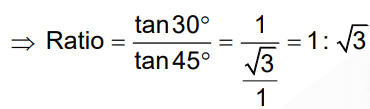Q.2. The ratio of the distances travelled by a freely falling body in the 1st, 2nd, 3rd, and 4th second       
(1) 1 : 4 : 9 : 16
(2) 1 : 3 : 5 : 7
(3) 1 : 1 : 1 : 1
(4) 1 : 2 : 3 : 4
Ans: (2)
Solution: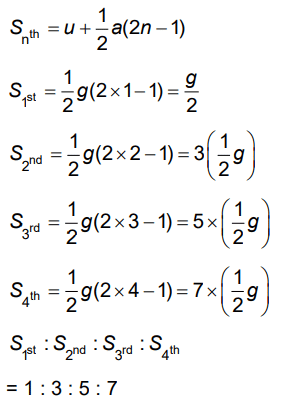Q.3. A small block slides down on a smooth inclined plane starting from rest at time t=0. Let Sn be the distance travelled by the block in the interval t= n-1 to t=n. Then the ratio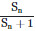is:       
A: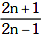B: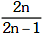C: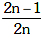D: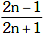Ans: D
Solution:
Sn = Distance in nth sec.
i.e. t = n – 1 to t = n
Sn + 1 = Distance in (n + 1)th sec.
i.e. t = n to t = n + 1
So as we know,Q.4. A ball is thrown vertically downward with a velocity of 20m/s from the top of a tower. It hits the ground after some time with a velocity of 80m/s. The height of the tower is : (g = 10m/s2)       
A:  320 m
B: 300 m
C: 360 m
D: 340 m
Ans: B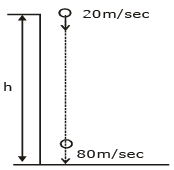v2 – u2 = 2as
(80)2  – (–20)2 = 2(–10) × s
6400 – 400 = 2 × (–10) × s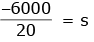s = – 300 m
Height of tower h = 300 m

Q.5. Preeti reached the metro station and found that the escalator was not working. She walked up the stationary escalator in time t1. On other days, if she remains stationary on the moving escalator, then the escalator takes her up in time t2. The time taken by her to walk up on the moving escalator will be     
A: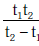B: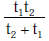C: t1 – t2
D: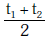Ans:
B
Solution:
As we learnt in
Case of Relative velocity -
When A & B are moving along with straight line in opposite direction.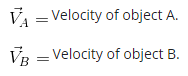Relative velocity of A with respect to B is.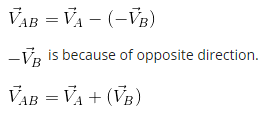Q.6. A particle moves so that its position vector is given by r = cosωtx + sinωty. Where ω is a constant. Which of the following is true?
A: Velocity is perpendicular to r and acceleration is directed away from the origin.
B: Velocity and acceleration both are perpendicular to r
C: Velocity is acceleration both are parallel to r
D: Velocity is perpendicular to r and acceleration is directed towards the origin.    
Ans:
D
Solution: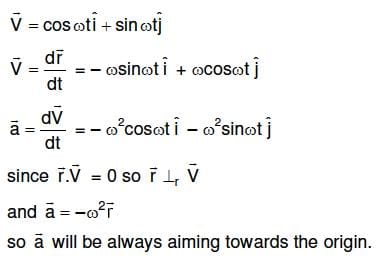Q.7. If the velocity of a particle is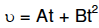, where A and B are constants, then the distance travelled by it between 1s and 2s is    
A: A/2 + B/3
B: 3A/2 + B
C: 3A + 7B
D: 3A/2 + 7B/3
Ans:
D
Solution: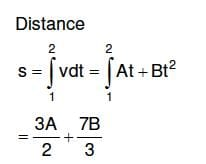Q.8. A particle of unit mass undergoes one-dimensional motion such that its velocity varies according to v(x) = βx-2n, where β and n are constants and x is the position of the particle. The acceleration of the particle as a function of x, is given by :    
A: -2nβ2 e-4n + 1
B: -2nβ2 x-2n - 1
C: -2nβ2 x-4n - 1
D: -2nβ2 x-2n + 1
Ans:
C
Solution: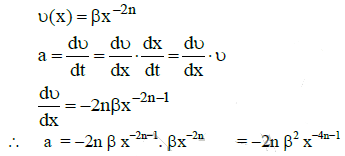The document Previous Year Questions (2014-22): Motion in a Straight Line - Notes | Study Physics Class 11 - NEET is a part of the NEET Course Physics Class 11.
All you need of NEET at this link: NEET

## Physics Class 11

127 videos|464 docs|210 tests
 Use Code STAYHOME200 and get INR 200 additional OFF

## Physics Class 11

127 videos|464 docs|210 tests

### How to Prepare for NEET

Read our guide to prepare for NEET which is created by Toppers & the best Teachers

Track your progress, build streaks, highlight & save important lessons and more!

,

,

,

,

,

,

,

,

,

,

,

,

,

,

,

,

,

,

,

,

,

;